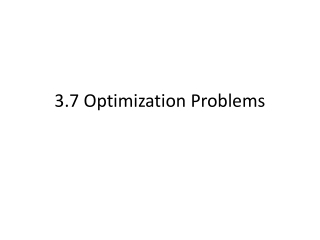DownloadDownload Presentation3.7 Optimization Problems

# 3.7 Optimization Problems

Download Presentation## 3.7 Optimization Problems

- - - - - - - - - - - - - - - - - - - - - - - - - - - E N D - - - - - - - - - - - - - - - - - - - - - - - - - - -
##### Presentation Transcript

1. 3.7 Optimization Problems

2. Finding Maximum Volume • A manufacturer wants to design an open box having a square base and a surface area of 108 square inches. What dimensions will produce a box with maximum volume?

3. Because the box has a square base, its volume is • Surface area of the box is

4. Because we are trying to maximize volume…..

5. Since x cannot be negative, we use 6. • You can check it by taking the second derivative test.

6. Finding Minimum Distance • Which points on the graph of are closest to the point ?

7. Finding Minimum Distance Using the equation, • Because d is the smallest when the expression inside the radical is smallest, you need to find the critical numbers of

8. Finding Minimum Area • A rectangular page is to contain 24 square inches of print. The margins at the top and bottom of the page are to be 1.5 inches, and the margins on the left and right are to be 1 inch. What should the dimensions of the page be so that the least amount of paper is used?

9. Let A be the area to be minimized

10. Finding Mimimum Length • Two posts, one 12 feet high and the other 28 feet high, stand 30 feet apart. They are to be stayed by two wires, attached to a single stake, running from ground level to the top of each post. Where should the stake be placed to use the least amount of wire?

11. Let W be the wire length to be minimized.

12. So, W is

13. An Endpoint Maximum Four feet of wire is to be used to form a square and a circle. How much of the wire should be used for the circle to enclose the maximum total area?

14. A=(area of square) + (area of circle) Because the total length of wire is 4 feet, So, then……

15. An Endpoint Maximum continued The only critical number in (0,1) is So, We can conclude that the maximum area occurs when x=0. That is, all the wire is used for the circle.